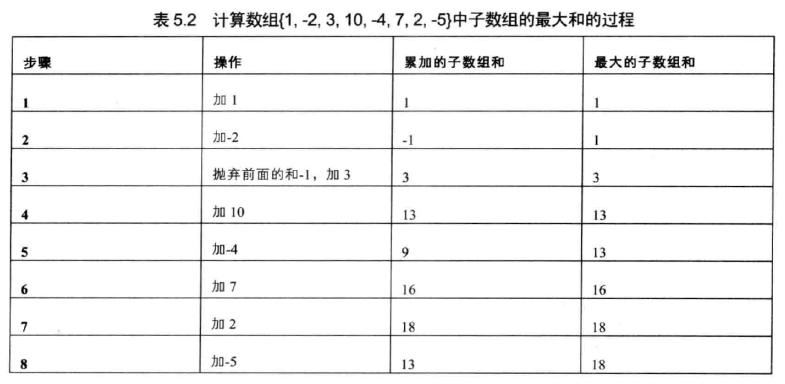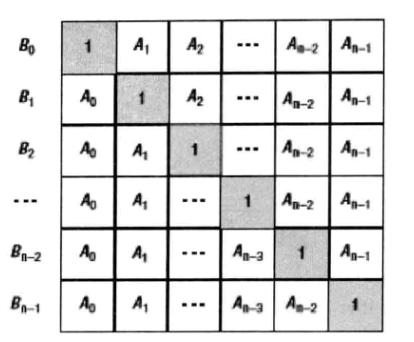# 《剑指Offer》——数组（Python3 实现）

1、二维数组中的查找

2、旋转数组的最小数字

3、调整数组顺序使奇数位于偶数前面

4、数组中出现次数超过一半的数字

5、连续子数组的最大和

6、把数组排成最小的数

7、数字在排序数组中出现的次数

8、数组中只出现一次的数字

9、数组中重复的数字

10、构建乘积数组

### 1、二维数组中的查找

# -*- coding:utf-8 -*-
class Solution:
# array 二维列表
def Find(self, target, array):
if array==[[]]:  #不能用not array，因为[[]]不为空，它是有一个元素为列表，但列表为空的数组
return False
x=len(array)-1
y=len(array)-1
#从左下角开始遍历
startX=0
startY=y
#循环条件，到右上点结束，因为查找方向为向右或向上
while startX<=x and startY>=0:
if array[startX][startY]>target:
startY-=1
elif array[startX][startY]<target:
startX+=1
else:
return True
return False

# -*- coding:utf-8 -*-
class Solution:
# array 二维列表
def Find(self, target, array):
# write code here
rows=len(array)
cols=len(array)
for i in range(rows):
for j in range(cols):
if target==array[i][j]:
return True
return False
if __name__=='__main__':
arr=[[1,2,3],[7,8,9],[11,12,13]]
tar=9
print(answer.Find(tar,arr))

### 2、旋转数组的最小数字

# -*- coding:utf-8 -*-
class Solution:
def minNumberInRotateArray(self, rotateArray):
start=0
end=len(rotateArray)-1
while start<end:
mid=(start+end)//2
#中间值<最左边值，由于本身是有两个递增数组组成，所以中间值右边的不需要考虑
if rotateArray[mid]<rotateArray[start]:
end=mid
elif rotateArray[mid]>rotateArray[start]:
start=mid
else:
return rotateArray[mid+1]

### 3、调整数组顺序使奇数位于偶数前面

# -*- coding:utf-8 -*-
class Solution:
def reOrderArray(self,array):
if not array:
return []
odd=[]
even=[]
for i in array:
if i%2==1:
odd.append(i)
else:
even.append(i)
return odd+even

### 4、数组中出现次数超过一半的数字

# -*- coding:utf-8 -*-
class Solution:
def MoreThanHalfNum_Solution(self, numbers):
# write code here
lenth=len(numbers)
a=set(numbers)
for i in a:
if numbers.count(i)>lenth/2:
return i
return 0

### 5、连续子数组的最大和# -*- coding:utf-8 -*-
class Solution:
def FindGreatestSumOfSubArray(self,array):
if not array:
return []
sum_arr=0
max_sum=array
for i in array:
if sum_arr>0:
sum_arr+=i
else:
sum_arr=i
max_sum=max(sum_arr,max_sum)
return max_sum

if __name__=='__main__':
array=[1,-2,3,10,-4,7,2,-5]
result=Solution()
print(result.FindGreatestSumOfSubArray(array))

### 6、把数组排成最小的数

# -*- coding:utf-8 -*-
class Solution:
def cmp(self,x,y):  #比较左+右，右+左，判断哪个小，然后返回
s1=str(x)+str(y)
s2=str(y)+str(x)
if s1<=s2:
return s1
if s1>s2:
return s2

def PrintMinNumber(self,numbers):
if not numbers:
return ''
numbers.sort()  #由小到大排序
#不断替换index为0，1的值为cmp的返回值，直至最后数组中仅剩一个元素
while len(numbers)>1:
numbers=self.cmp(numbers,numbers)
numbers.remove(numbers)
return numbers

if __name__=='__main__':
array=[3,32,321]
result=Solution()
print(result.PrintMinNumber(array))


### 变形：把数组排成最大的数。

class Solution:
def cmp(self,x,y):  #比较左+右，右+左，判断哪个大，然后返回
s1=str(x)+str(y)
s2=str(y)+str(x)
if s1<=s2:
return s2
if s1>s2:
return s1

def PrintMinNumber(self,numbers):
if not numbers:
return ''
numbers.sort(reverse=True)  #由大到小排序
#不断替换index为0，1的值为cmp的返回值，直至最后数组中仅剩一个元素
while len(numbers)>1:
numbers=self.cmp(numbers,numbers)
numbers.remove(numbers)
return numbers


### 7、数字在排序数组中出现的次数

# -*- coding:utf-8 -*-
class Solution:
def GetNumberOfK(self, data, k):
# write code here
count=0
if not data or k not in data:
return 0
for i in data:
if i==k:
count+=1
return count

### 8、数组中只出现一次的数字

# -*- coding:utf-8 -*-
class Solution:
# 返回[a,b] 其中ab是出现一次的两个数字
def FindNumsAppearOnce(self, array):
# write code here
number=[]
for i in array:
if i in number:
number.remove(i)
else:
number.append(i)
return number

### 9、数组中重复的数字

# -*- coding:utf-8 -*-
class Solution:
# 这里要特别注意~找到任意重复的一个值并赋值到duplication
# 函数返回True/False
def duplicate(self, numbers, duplication):
# write code here
if numbers==None or numbers==[]:
return False
data=[]
for i in numbers:
if i in data:
duplication=i
return True
else:
data.append(i)
return False

### 10、构建乘积数组# -*- coding:utf-8 -*-
class Solution:
def multiply(self, A):
# write code here
if not A:
return []
#计算左三角
num=len(A)
B=[None]*num
B=1
for i in range(1,num):
B[i]=B[i-1]*A[i-1]
#计算右三角，自下而上
#保留上次的计算结果乘本轮新的数，因为只是后半部分进行累加，所以设置一个temp，能够保留上次的结果
temp=1
for i in range(num-2,-1,-1): #从后往前遍历，不算最后一个（num-1），第一个for循环已经计算了
temp*=A[i+1]
B[i]*=temp
return B

# -*- coding:utf-8 -*-
class Solution:
def multiply(self, A):
# write code here
n=len(A)
B=*n
for i in range(n):
for j in range(n):
if i!=j:
B[i]*=A[j]
return B

12-13456306-011751
10-26125
10-2245
11-0575
08-0590
11-0786
04-011102
04-08615
12-2028
04-21492
07-2923
04-07113
11-1694
07-303万+
06-29427
03-171538
07-21141
08-20